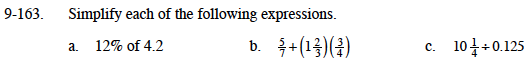### Home > MC1 > Chapter 9 > Lesson 9.3.4 > Problem9-163

9-163.Multiply 4.2 by 0.12 to find the answer.

0.504

Make sure to multiply before you add the terms.

$1\frac{27}{28}$

$\text{0.125 is equal to }\frac{1}{8} \text{ and 10 and one-fourth is equal to }\frac{41}{4}.$